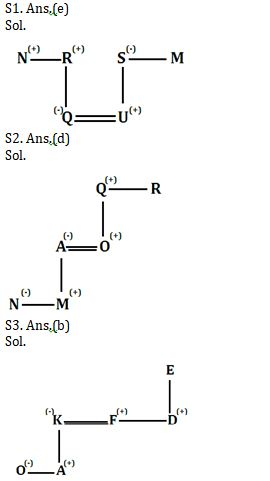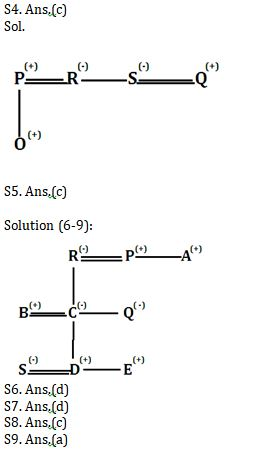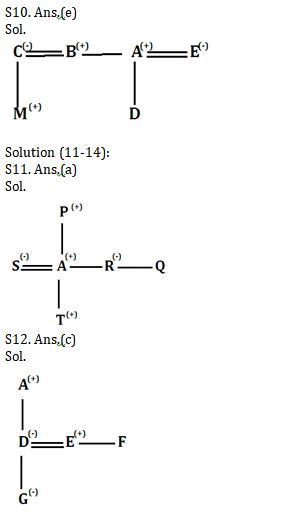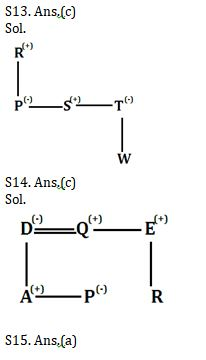Latest Banking jobs   »   Reasoning Ability, Direction Sense Quiz For...

# Reasoning Ability, Direction Sense Quiz For SBI PO, Clerk Prelims 2021- 29th June

Directions (1-4): Each of these questions is based on the following information:
(i) P % Q means P is the father of Q.
(ii) P @ Q means P is the sister of Q.
(iii) P \$ Q means P is the brother of Q.
(iv) P * Q means P is the son of Q.
(v) P # Q means P is the wife of Q.

Q1. In the expression N \$ R % Q # U * S @ M, how is Q related to N?
(a) Aunt
(b) Mother
(c) Sister
(d) Sister in law
(e) Niece

Q2. In the expression N @ M * A # O * Q \$ R, how is Q related to N?
(a) Aunt
(b) Uncle
(c) Sister
(d) Grand father
(e) Cousin

Q3. Which of the following relation shows ‘K is daughter in law of E’?
(a) F @ K \$ D % E # A * O
(b) O @ A * K # F \$ D * E
(c) A % O * K @ D # E \$ F
(d) K # D \$ A @ E * F % O
(e) None of these

Q4. Which of the following symbol is used if ‘O is nephew of S’ is true in the relation
P % O ____ R @ S # Q?
(a) #
(b) %
(c) *
(d) @
(e) \$

Q5. A man says to a lady, “Your father is the father in law of my father’s only child.” How is the lady related to the man?
(a) Sister-in-law
(b) Granddaughter
(c) Wife
(d) Daughter
(e) Aunt

Direction (6-9): Study the following information carefully and answer the given questions.
There are nine members in a family i.e. A, B, C, D, E, P, R, Q, and S. There are four females and three married couples. A is paternal uncle of Q. R has only 2 children. S is daughter-in-law of B. C is married to B. D and E are sons of C. E is not married. P is married to R. B is son-in-law of R. P is a male member.

Q6. Who among the following is brother in law of Q?
(a) E
(b) D
(c) P
(d) B
(e) None of these

Q7. Which of the following is true about P?
(a) P is uncle of Q
(b) B is daughter in law of P
(c) P is sister of A
(d) D is grandson of P
(e) P is father of E

Q8. How E is related to son in law of R?
(a) Sister in law
(b) Daughter
(c) Son
(d) Daughter in law
(e) Wife

Q9. How B’s sister in law is related to R’s brother in law?
(a) Niece
(b) Nephew
(c) Son
(d) Daughter
(e) Sister

Q10.C is the wife of B. M is the son of C. A is the brother of B and father of D. E is wife of A. How is B related to D?
(a) Sister
(b) Cousin
(c) Brother
(d) Mother
(e) Uncle

Directions (11-14): Each of these questions is based on the following information:
(i) A % B means A is the daughter of B.
(ii) A @ B means A is the mother of B.
(iii) A \$ B means A is the father of B.
(iv) A * B means A is the son of B.
(v) A © B means A is brother of B

Q11. Which of the following shows the relation that S is sister in law of Q?
(a) S @ T * A © R % P \$ Q
(b) S % A @ T * R © Q \$ P
(c) T @ S © R \$ Q % P
(d) R % S \$ P * Q
(e) None of these

Q12. Who among the following is F’s niece in this relation- ‘A \$ D @ G % E © F’?
(a) A
(b) D
(c) G
(d) E
(e) Can’t be determine

Q13. If the expression ‘P % R \$ S © T @ W’ true, then which of the following is true?
(a) P is brother of T
(b) S is daughter of R
(c) P is aunt of W
(d) R is grandmother of W
(e) None is true

Q14. If the expression ‘A * D @ P % Q © E \$ R’ true, then which of the following is false?
(a) E is uncle of A
(b) P is daughter of D
(c) Q is aunt of R
(d) E is brother in law of D
(e) All are true

Q15. Showing a man on the stage, Rita said, ” He is the brother of the daughter of the wife of my husband. How is the man on stage related to Rita?
(a) Son
(b) Husband
(c) Cousin
(d) Nephew
(e) Niece

Practice More Questions of Reasoning for Competitive Exams:

###### SBI Clerk Study Plan 2021

Solutions